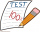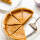# Fraction calculator

The calculator performs basic and advanced operations with fractions, expressions with fractions combined with integers, decimals, and mixed numbers. It also shows detailed step-by-step information about the fraction calculation procedure. Solve problems with two, three, or more fractions and numbers in one expression.

## Result:

### 51/4 * 13/8 = 231/32 = 7 7/32 = 7.21875

Spelled result in words is two hundred thirty-one thirty-seconds (or seven and seven thirty-seconds).

### How do you solve fractions step by step?

1. Conversion a mixed number 5 1/4 to a improper fraction: 5 1/4 = 5 1/4 = 5 · 4 + 1/4 = 20 + 1/4 = 21/4

To find new numerator:
a) Multiply the whole number 5 by the denominator 4. Whole number 5 equally 5 * 4/4 = 20/4
b) Add the answer from previous step 20 to the numerator 1. New numerator is 20 + 1 = 21
c) Write a previous answer (new numerator 21) over the denominator 4.

Five and one quarter is twenty-one quarters
2. Conversion a mixed number 1 3/8 to a improper fraction: 1 3/8 = 1 3/8 = 1 · 8 + 3/8 = 8 + 3/8 = 11/8

To find new numerator:
a) Multiply the whole number 1 by the denominator 8. Whole number 1 equally 1 * 8/8 = 8/8
b) Add the answer from previous step 8 to the numerator 3. New numerator is 8 + 3 = 11
c) Write a previous answer (new numerator 11) over the denominator 8.

One and three eighths is eleven eighths
3. Multiple: 21/4 * 11/8 = 21 · 11/4 · 8 = 231/32
Multiply both numerators and denominators. Result fraction keep to lowest possible denominator GCD(231, 32) = 1. In the next intermediate step, the fraction result cannot be further simplified by canceling.
In words - twenty-one quarters multiplied by eleven eighths = two hundred thirty-one thirty-seconds.

#### Rules for expressions with fractions:

Fractions - use the slash “/” between the numerator and denominator, i.e., for five-hundredths, enter 5/100. If you are using mixed numbers, be sure to leave a single space between the whole and fraction part.
The slash separates the numerator (number above a fraction line) and denominator (number below).

Mixed numerals (mixed fractions or mixed numbers) write as non-zero integer separated by one space and fraction i.e., 1 2/3 (having the same sign). An example of a negative mixed fraction: -5 1/2.
Because slash is both signs for fraction line and division, we recommended use colon (:) as the operator of division fractions i.e., 1/2 : 3.

Decimals (decimal numbers) enter with a decimal point . and they are automatically converted to fractions - i.e. 1.45.

The colon : and slash / is the symbol of division. Can be used to divide mixed numbers 1 2/3 : 4 3/8 or can be used for write complex fractions i.e. 1/2 : 1/3.
An asterisk * or × is the symbol for multiplication.
Plus + is addition, minus sign - is subtraction and ()[] is mathematical parentheses.
The exponentiation/power symbol is ^ - for example: (7/8-4/5)^2 = (7/8-4/5)2

#### Examples:

subtracting fractions: 2/3 - 1/2
multiplying fractions: 7/8 * 3/9
dividing Fractions: 1/2 : 3/4
exponentiation of fraction: 3/5^3
fractional exponents: 16 ^ 1/2
adding fractions and mixed numbers: 8/5 + 6 2/7
dividing integer and fraction: 5 ÷ 1/2
complex fractions: 5/8 : 2 2/3
decimal to fraction: 0.625
Fraction to Decimal: 1/4
Fraction to Percent: 1/8 %
comparing fractions: 1/4 2/3
multiplying a fraction by a whole number: 6 * 3/4
square root of a fraction: sqrt(1/16)
reducing or simplifying the fraction (simplification) - dividing the numerator and denominator of a fraction by the same non-zero number - equivalent fraction: 4/22
expression with brackets: 1/3 * (1/2 - 3 3/8)
compound fraction: 3/4 of 5/7
fractions multiple: 2/3 of 3/5
divide to find the quotient: 3/5 ÷ 2/3

The calculator follows well-known rules for order of operations. The most common mnemonics for remembering this order of operations are:
PEMDAS - Parentheses, Exponents, Multiplication, Division, Addition, Subtraction.
BEDMAS - Brackets, Exponents, Division, Multiplication, Addition, Subtraction
BODMAS - Brackets, Of or Order, Division, Multiplication, Addition, Subtraction.
GEMDAS - Grouping Symbols - brackets (){}, Exponents, Multiplication, Division, Addition, Subtraction.
Be careful, always do multiplication and division before addition and subtraction. Some operators (+ and -) and (* and /) has the same priority and then must evaluate from left to right.

## Fractions in word problems:

• Ten fractionsWrite ten fractions between 1/3 and 2/3
• Stones in aquariumIn an aquarium with a length of 2 m, 1.5 m wide, and 2.5 m deep, the water is up to three-quarters of the depth. Can we place 2m cubic meters of stones in the aquarium without spilling water? (0 = no, 1 = yes)
• TorqueTorque and Mari each multiplied 1/8 inch times 5/8 inch. Tartaric 5/8 squares point inches. And Marie got 5/64 squared thought inches tall. Which student found a corrupt area?Rhea answered 5/11 in the questions correctly and Precious answered 7/11 of it correctly. If each problem is worth the same amount, who got the higher score?
• Turtles 2A box turtle hibernates in the sand at 11 5/8. A spotted turtle hibernates at 11 16/25 feet. Which turtle is deeper? Write answer as number 1 or 2.
• Stones in aquariumIn an aquarium with a length 2 m; width 1.5 m and a depth of 2.5 m is a water level up to three-quarters of the depth. Can we place stones with a volume of 2 m3 into the aquarium without water being poured out?
• Giraffes to monkeysThe ratio of the number of giraffes to the number of monkeys in a zoo is 2 to 5. Which statement about the giraffes and monkeys could be true? A. For every 10 monkeys in the zoo, there are 4 giraffes. B. For every  giraffe in the zoo, there are 3 monkeys.
• Math testBrayden was solving some math problems for the math team. He answered 2 math problems. Matthew answered 3, John answered 1 reasoning. Matthew 1/2 times as many. Brayden said that 2/6. Is he correct? Why or why not? Be sure to explain your answer.
• Pumpkin pieHave some pumpkin pie. One half of the pie is cut into 4 equal slices, the other half is cut into 3 equal slices. After eating one of the larger slices (on the 3 piece side), I wonder if I will eat more if I have one more from the 3 side, or two from the
• Engineer KažimírThe difference between politicians-demagogues and reasonable person with at least primary education beautifully illustrated by the TV show example. "Engineer" Kažimír says that during their tenure there was a large decline in the price of natural gas, pri
• Roma ateRoma ate 2/5 of a cake while Somya ate 3/7 of the same cake. Who ate more and by how much?
• SandySandy, John and Marg baked pies for the Bake Sale. Sandy cut his pies into 6ths, John but his into 8ths and Marg cut hers into quarters. Sandy sold 11/6, John sold  1 3/8 pies and Marg sold 9/4 pies. Who sold the most pies? Who sold the fewest?
• Colored blocksTucker and his classmates placed colored blocks on a scale during a science lab. The brown block weighed 8.94 pounds, and the red block weighed 1.87 pounds. How much more did the brown block weigh than the red block?